# Tank

(To be removed) Pressurized fluid container with variable fluid level

The Hydraulics (Isothermal) library will be removed in a future release. Use the Isothermal Liquid library instead. (since R2020a)

•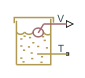Libraries:
Simscape / Fluids / Hydraulics (Isothermal) / Low-Pressure Blocks

## Description

The Tank block models a pressurized fluid container with a variable fluid level. The tank has an optional number of ports ranging from one to three, with each number corresponding to a block variant. The tank pressurization is fixed regardless of changes to fluid volume or fluid level. The tank is vented if the pressurization is set to zero—a setting corresponding to an internal pressure equal to atmospheric pressure.

Tank Ports and Port Elevations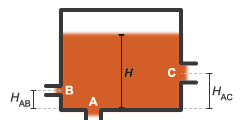The block accounts for differences in port elevation. Hydrostatic pressure is computed separately for each port from the fluid level and port elevation. The hydrostatic pressure adds to the pressurization of the tank—increasing the port pressure as the fluid level rises. The block accounts also for minor pressure losses at the ports due to filters, fittings, and other local flow resistances. To modulate these losses, the block provides a pressure loss coefficient for each port.

### Changing the Number of Ports

To change the number of ports in the tank, use the parameter to change the active block variant. Variant options include:

• `One inlet` (default) — Exposes one hydraulic conserving port, .

• `Two inlets` — Exposes hydraulic conserving ports A and .

• `Three inlets` — Exposes hydraulic conserving ports A, B, and C.

Port A in the `Two inlets` and `Three inlets` variants is a direct replacement of port T in the `One inlet` variant.

### Port Depth and Fluid Level

Port T in the `One inlet` variant—or port A, its replacement in the `Two inlets` and ```Three inlets``` variants—is by default located at the bottom of the tank. The depth of this port relative to the top of the fluid volume is equal to the fluid level—the height of the fluid volume:

`${y}_{T/A}=H,$`

where:

• yT/A is the depth of port T or A.

• H is the fluid level in the tank.

Ports B and C in the ```Two inlets``` and `Three inlets` variants are assumed to be located above port A. The depths of these ports are each computed as the difference between the fluid level and the port elevation above port A:

`${y}_{B}=H-{H}_{AB},$`

and

`${y}_{C}=H-{H}_{AC},$`

where:

• yB and yC are the depths of ports B, and C.

• HAB and HAC are the elevations of ports B and C above port A.

The fluid level, H, is computed during simulation from the instantaneous fluid volume. This calculation depends on the Tank volume parameterization setting. If the parameterization is set to `Constant cross-sectional area` (the default setting), the fluid level is:

`$H=\frac{V}{S},$`

where:

• V is the instantaneous fluid volume in the tank.

• S is the internal cross-sectional area of the tank, specified in the Tank cross-section area parameter.

If the Tank volume parameterization is set to `Tabulated data — Volume vs. level`, the fluid level is computed by interpolation or extrapolation of tabulated fluid level data specified as a function of fluid volume:

`$H=f\left(V\right)$`

The instantaneous fluid volume varies with the volumetric flow rates through the exposed ports. This volume increases if the sum of all flow rates is positive. A flow rate is positive if it is directed from the port to the interior of the tank, that is, if fluid enters the tank. The time rate of change of the fluid volume is defined as:

`$\frac{dV}{dt}=\sum _{i}{q}_{i},$`

where qi is the flow rate into the tank through the port denoted by iT/A, B, or C.

### Flow Rate and Pressure Drop

The volumetric flow rate through a port is a function of the pressure drop from that port to the interior of the tank. The flow rate is positive if the pressure drop is positive—that is, if pressure is higher at the port than in the tank—and negative otherwise:

`${q}_{i}={A}_{i}\sqrt{\frac{2}{{K}_{i}\rho }}\frac{\Delta {p}_{i}}{{\left(\Delta {p}_{i}^{2}+{p}_{Cr,i}^{2}\right)}^{1/4}},$`

where:

• Ai is the internal cross-sectional area of the port denoted by i (T/A, , or C):

`${A}_{i}=\frac{\pi {d}_{i}^{2}}{4},$`

with di as the internal diameter of the port.

• Ki is the pressure loss coefficient specified for the port.

• ρ is the hydraulic fluid density.

• Δpi is the pressure drop from the port to the interior of the tank.

• pCr,i is the critical pressure at which the flow through the port switches between laminar and turbulent.

The critical pressure at a port is computed from the critical Reynolds number, internally set to a value of `15`, and from the respective port diameter. The critical pressure is modulated by the pressure loss coefficient specified for the port—with this parameter serving as a gain for the critical pressure:

`${p}_{Cr,i}={K}_{i}\frac{\rho }{2}\left(\frac{{\mathrm{Re}}_{Cr}\nu }{{d}_{i}}\right),$`

where:

• ReCr is the critical Reynolds number.

• ν (nu) is the hydraulic fluid dynamic viscosity.

• di is the internal diameter of the port denoted by i.

The pressure drop from a port to the interior of the tank is computed from the specified Pressurization value, the hydrostatic pressure, and the pressure at the port:

`$\Delta {p}_{i}={p}_{port,i}-\left({p}_{press}+{p}_{elev,i}\right),$`

where:

• pport,i is the pressure at the port denoted by i.

• ppress is the specified tank Pressurization value.

• pelev,i is the hydrostatic pressure at the port.

The hydrostatic pressure at a port is a function of the port elevation above the bottom of the tank (zero for port T/A):

`${p}_{elev,i}=\rho g{y}_{i},$`

where

• g is the value of the gravitational acceleration at the mean elevation of the system.

• yi is the elevation of the port denoted by i relative to the bottom of the port.

### C/C++ Code Generation

This block supports code generation for real-time simulation tasks. Certain blocks and block settings may be more suitable for simulation on a real-time device. For suggestions on how to improve real-time simulation performance, use the Simulink® Performance Advisor function. Suggestions include ways to reduce model complexity and decrease numerical stiffness.

In the Simulink Editor, click the Debug tab and select Performance Advisorfrom Performance Advisor. Set the Activity parameter to ```Execute real-time application``` to view suggestions specific to real-time simulation performance. Expand the Real-Time node in the tree view pane to select performance checks specific to Simscape™ products.

### Variables

To set the priority and initial target values for the block variables prior to simulation, use the Initial Targets section in the block dialog box or Property Inspector. For more information, see Set Priority and Initial Target for Block Variables.

Nominal values provide a way to specify the expected magnitude of a variable in a model. Using system scaling based on nominal values increases the simulation robustness. Nominal values can come from different sources, one of which is the Nominal Values section in the block dialog box or Property Inspector. For more information, see Modify Nominal Values for a Block Variable.

## Ports

### Output

expand all

Physical signal output port reporting the volume of fluid in the tank.

### Conserving

expand all

Hydraulic (isothermal liquid) conserving port representing the tank inlet.

#### Dependencies

To enable this port, set Modeling option to `One inlet`.

Hydraulic (isothermal liquid) conserving port representing one of multiple tank inlets.

Hydraulic (isothermal liquid) conserving port representing one of multiple tank inlets.

#### Dependencies

To enable this port, set Modeling option to `Two inlets` or `Three inlets`.

Hydraulic (isothermal liquid) conserving port representing one of multiple tank inlets.

#### Dependencies

To enable this port, set Modeling option to `Three inlets`.

## Parameters

expand all

Set the number of ports for the block.

### Main

Gauge pressure of the tank volume. The tank pressure is held constant at this value during simulation. The default value, `0` Pa, corresponds to a vented tank—one whose internal pressure is equal to the atmospheric pressure of the system.

Choice of parameterization for the tank volume. Select `Constant cross-sectional area` to compute the tank volume from the variable fluid level and a constant cross-sectional area. Select ```Tabulated data — volume vs. level``` to compute the tank volume by interpolation or extrapolation of tabulated data on the fluid volume at discrete fluid levels.

Area of the tank cross-section, assumed constant over the allowed range of fluid levels. The block uses this parameter to compute the volume of fluid inside the tank.

Array of fluid volumes for the 1-D lookup table used to compute the tank fluid level. The array must increase from left to right but the intervals between the array element values need not be uniform. There must be at least two elements for `Linear` interpolation and three elements for `Smooth` interpolation.

#### Dependencies

This parameter is active when the Tank volume parameterization parameter is set to ```Tabulated data — volume vs. level```.

Array of fluid levels corresponding to the specified orifice openings. The number of elements in the array must match the number of elements in the Opening vector parameter.

#### Dependencies

This parameter is active when the Tank volume parameterization parameter is set to ```Tabulated data — volume vs. level```.

Method of computing values inside the tabulated data range. The `Linear` method joins adjacent data points with straight line or surface segments with generally discontinuous slope at the segment boundaries. Surface segments are used in the 2-D lookup table specified in the ```Pressure-flow characteristic``` model parameterization.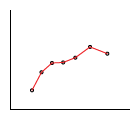The `Smooth` method replaces the straight segments with curved versions that have continuous slope everywhere inside the tabulated data range. The segments form a smooth line or surface passing through all of the tabulated data points without the discontinuities in first-order derivatives characteristic of the `Linear` interpolation method.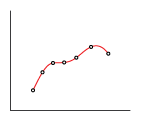#### Dependencies

This parameter is active when the Tank volume parameterization parameter is set to ```Tabulated data — Volume vs. level```.

Method of computing values outside of the tabulated data range. The `Linear` method extends the line segment drawn between the last two data points at each end of the data range outward with a constant slope.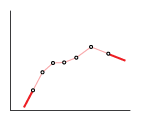The `Nearest` method extends the last data point at each end of the data range outward as a horizontal line with constant value.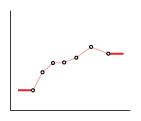#### Dependencies

This parameter is active when the Tank volume parameterization parameter is set to ```Tabulated data — Volume vs. level```.

Diameter of the tank inlet. The tank has a single port when this parameter is exposed.

#### Dependencies

This parameter is active when the block variant is set to `One inlet`.

Diameter of tank port A/B/C.

#### Dependencies

This parameter is active when the block variant is set to `Two inlets` or ```Three inlets```.

Empirical coefficient used to account for pressure losses at the tank inlet. This parameter must be greater than zero. The block has a single port when this parameter is exposed.

#### Dependencies

This parameter is active when the block variant is set to `One inlet`.

Empirical coefficient used to account for pressure losses at port A/B/C. This parameter must be greater than zero.

#### Dependencies

This parameter is active when the block variant is set to `Two inlets` or ```Three inlets```.

Elevation of port B/C above port A. The block uses this parameter to account for differences in port elevation.

#### Dependencies

This parameter is active when the block variant is set to `Two inlets` or ```Three inlets```.

Gravitational acceleration constant at the mean elevation of the hydraulic system. Changes in gravitational acceleration are assumed negligible over the height of the system.

Lowest fluid level allowed during simulation. The block triggers a simulation warning if the fluid level falls below the specified value.

Simulation warning mode for invalid fluid levels in the tank. Select `Warning` to be notified when the fluid level falls below a minimum specified value.

## Version History

collapse all

### R2023a: To be removed

The Hydraulics (Isothermal) library will be removed in a future release. Use the Isothermal Liquid library instead.